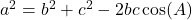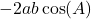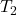# The Cosine Rule

The cosine rule is used to calculate angles and sides on a triangle. It can be used on right angle triangles or non-right angle triangles.

The cosine rule can be used to calculate a side when one angle and its two adjacent sides are given:

The cosine rule can be used to calculate an angle when all three sides are given:

The cosine rule is similar to the Pythagorean Theorem, however the Pythagorean Theorem can only be used with right angled triangles.

According the Pythagorean Theorem, when the angle at vertex A in the figure above is exactly 90 degrees, thenUse the slider in the applet below to change the angle at vertex A.

Notice that by changing the angle, the valuedoesn’t change, but the value ofchanges.

The cosine rule tells us how to calculate the valuefor any triangle:

To find a side lengthon triangle ABC,Recall (from the Unit Circle) that, therefore for a right angle triangle. At 90 degrees, the cosine rule says the same as the Pythagorean Theorem.

Recall also that in the second quadrant (obtuse angles) cosine is negative. Therefore for obtuse angle triangles,is a positive value, makinggreater than. This agrees with the applet above.

We’ll find out exactly wherecomes from after the examples.

# Example 1: Calculate the third side

We can use the cosine rule to calculate the third side in this triangle.

In the formula, sidemust be opposite angle. The other two sides ‘b’ and the ‘c’ are interchangeable – it doesn’t matter which one is which.The remaining two angles can be calculated using the sine law or using the angle form of the cosine law discussed below.

# Example 2: Isosceles Triangle

Noticing that this is an isosceles triangle. Let’s fill in the side length that we know:

We’re now ready to use the cosine rule:# Calculate an Angle

Let’s rearrange the formula to isolate:This is the second form of the cosine rule.

To find an angleon triangle ABC,# Example 3: Calculate an Angle

What’s important to get right when using the cosine rule is that the side referred to asand the angle referred to asin the formula must be opposite each other.The next angle can be found using the cosine law again, or by using the sine law.

# Practice

Find online practice the cosine law here on transum math

Find worksheet practice on corbett maths. Note the QR code at the end of the exercise for solutions.

# Proofs of the Cosine Law

Here is a standard proof for high school. It is standard because it depends on the Pythagorean Theorem, SOH CAH TOA and some algebra.

Here is a link to a page on Cut The Knot that discusses the Cosine Law.

Check out this ‘proof without words.’

Here are just a few words in case they’re helpful….

Take any triangle, label the sides a, b, c. This is ‘First Triangle’

Enlarge ‘First Triangle’ by scale factor a, so now we have a triangle with side lengths aa, ab, and ac. This is.

Enlarge ‘First Triangle’ by scale factor b, so now we have a triangle with side lengths ab, bb, and bc. This is.

Enlarge ‘First Triangle’ by scale factor c, so now we have a triangle with side lengths ac, bc, and cc. This is.

Cut out,andand arrange them so that their equal sides are next to each other – to form a trapezoid.